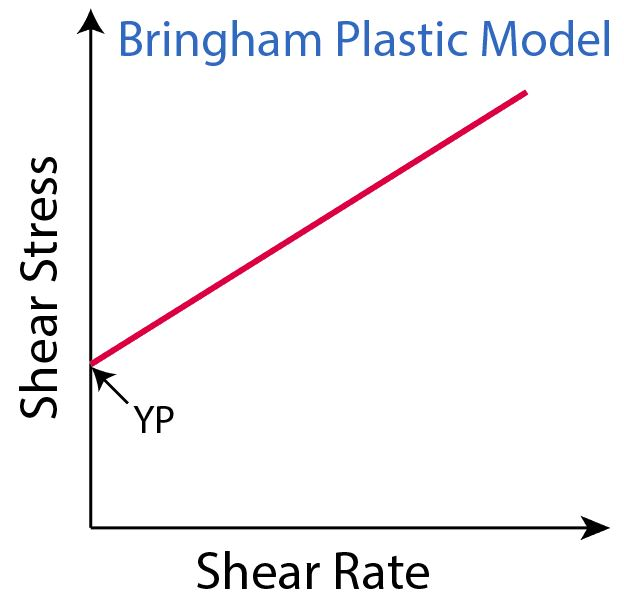## Yield Point (YP) of Drilling FluidsYield Point (YP) is resistance of initial flow of fluid or the stress required in order to move the fluid. It can be simply stated that the Yield Point (YP) is the attractive force among colloidal particles in drilling fluid. As per Bingham plastic model, YP is the shear stress extrapolated to a shear rate of zero.Figure 1 – YP is a shear stress at zero shear rate. Continue reading